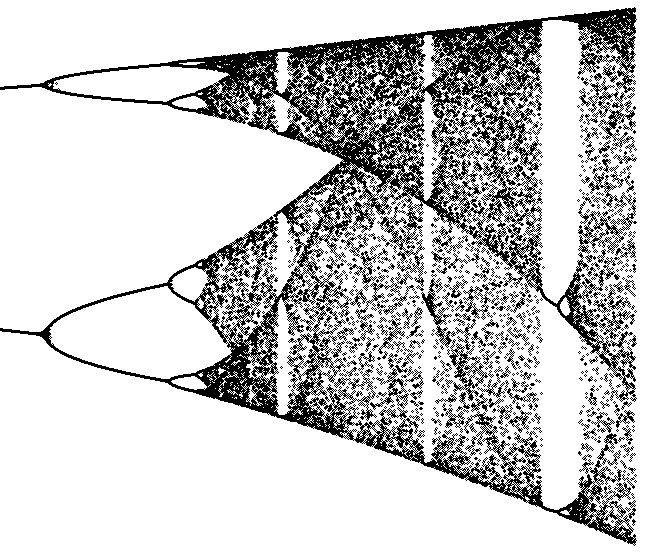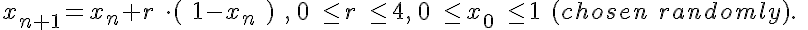# Logistic

Model number
0339

A n iterative procedure is used to draw the Logistic Map.Figure 1: The logistic map.

## Description

```The sequence given by

x(n+1) = r * x(n) * ( 1 - x(n) ), x(0) = random()
0<=r<=4

where random() is a uniform random number between 0 and 1
can be used to generate the Logistic Map. The x values
after 200 iterations are plotted as a function of r to
produce the logistic map.

For r = 2.8, the process converges to a single value.
For r = 3.3, the process oscillates between two values.
For r = 3.5, the process oscillates between four values.
For r = 3.8, the process is chaotic even though it is deterministic.

Plotting pairs of points ( x(n), x(n+1) ) reveals a one
dimensional curve (parabola). The logistic map is a fractal
because if any part of it is expanded, it reveals a similar
structure.

The logistic map shows bifurcating behavior. Small changes in
r can change the set of x values from a finite number to an
infinite number.

There are other variations of this algorithm which are
also called the Logistic equation, e.g.

x(n+1) = x(n) + r * x(n) * ( 1 - x(n) ), x(0) = random()
0<=r<=3.

```

## Equations

#### Iterative equation for Logistic MapThe equations for this model may be viewed by running the JSim model applet and clicking on the Source tab at the bottom left of JSim's Run Time graphical user interface. The equations are written in JSim's Mathematical Modeling Language (MML). See the Introduction to MML and the MML Reference Manual. Additional documentation for MML can be found by using the search option at the Physiome home page.

References
```Bassingthwaighte JB, Liebovitch LS, and West BJ. Fractal Physiology.
New York, London: Oxford University Press, 1994, 364 pp.

```
Key terms
Logistic
chaos
bifurcation
fractal
Acknowledgements

Please cite https://www.imagwiki.nibib.nih.gov/physiome in any publication for which this software is used and send one reprint to the address given below:
The National Simulation Resource, Director J. B. Bassingthwaighte, Department of Bioengineering, University of Washington, Seattle WA 98195-5061.

Model development and archiving support at https://www.imagwiki.nibib.nih.gov/physiome provided by the following grants: NIH U01HL122199 Analyzing the Cardiac Power Grid, 09/15/2015 - 05/31/2020, NIH/NIBIB BE08407 Software Integration, JSim and SBW 6/1/09-5/31/13; NIH/NHLBI T15 HL88516-01 Modeling for Heart, Lung and Blood: From Cell to Organ, 4/1/07-3/31/11; NSF BES-0506477 Adaptive Multi-Scale Model Simulation, 8/15/05-7/31/08; NIH/NHLBI R01 HL073598 Core 3: 3D Imaging and Computer Modeling of the Respiratory Tract, 9/1/04-8/31/09; as well as prior support from NIH/NCRR P41 RR01243 Simulation Resource in Circulatory Mass Transport and Exchange, 12/1/1980-11/30/01 and NIH/NIBIB R01 EB001973 JSim: A Simulation Analysis Platform, 3/1/02-2/28/07.